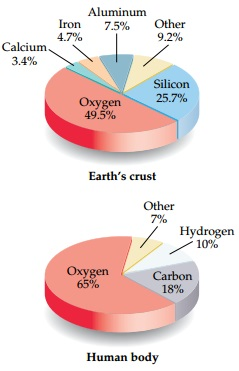# Problem: If the lower pie chart was drawn as the percentage in terms of number of atoms rather than the percentage in terms of mass, would the hydrogen slice of the pie get larger or smaller?

###### FREE Expert Solution

Avogadro’s number = 6.022 x 1023 entities

entities = atoms, ions, molecules, particles, formula units

Assuming total mass = 100 g then: % element = mass element

Solving for atoms of each element except others:

= 6.022 x 1023 H atoms

= 2.44 x 1024 O atoms

= 9.033 x 1023 O atoms

Use formula for each element:

% atom H = ~ 15.3 % > 10%

% atom O = ~ 61.8 %

# atom C = ~ 22.9 %

81% (185 ratings)###### Problem Details

If the lower pie chart was drawn as the percentage in terms of number of atoms rather than the percentage in terms of mass, would the hydrogen slice of the pie get larger or smaller?